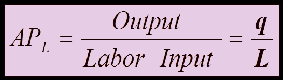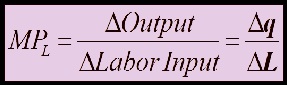Select Page

After the basic concept of Production Function and Production Decision. We now move on to Production techniques with one variable or you can also name it as Production Techniques in the Short-Run.

One of the Most basic Example of Inputs of Production used is the Capital and Labor, considering Labor as a variable input and Capital as fixed.

To increase the amount of Production in the Short Run with a Fixed Input of Capital , we increase the amount of labor required to produce additional Output. But to how much extent ? For example if there are 3 machine and 3 labor already working. Increasing the Labor to 6 would increase the Output but what if you hire more to 10 ? Probably 6 can work better over the equipment rather than 10. This also brings up few questions in mind

1. How much output is produced by each labor?
2. How much Output is produced after hiring additional Labor?

## Average and Marginal Products

About the above questions we have asked provides an explanation to both of these concepts.

Average Product is the Output Produced by per unit of an input.

So the average product of the variable input LABOR tells us how much output is produced by each labor on average.

Marginal Product is the additional output produced by one unit increase in Input (labor).

Marginal product tells us how much output is produced by hiring an additional labor. Infact ! it tells us that is it really worth having an additional labor or not ? This leads us to Diminishing Marginal Returns which we will discuss in the up coming topics. (See Law of Diminishing Returns)

### Mathematical Explanation of Average and Marginal Product

Taking labor as a variable input, it can be written mathematically as:

Average Product of Labor:Marginal Product of Labor:Upnext: The Slopes of Marginal and Average Product Curve.

Shares